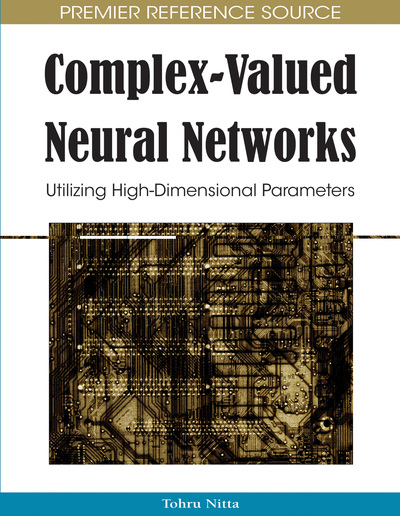# Attractors and Energy Spectrum of Neural Structures Based on the Model of the Quantum Harmonic Oscillator

G.G. Rigatos, S.G. Tzafestas
DOI: 10.4018/978-1-60566-214-5.ch015
OnDemand:
(Individual Chapters)
Available
\$37.50
No Current Special Offers

## Abstract

Neural computation based on principles of quantum mechanics can provide improved models of memory processes and brain functioning and is of primary importance for the realization of quantum computing machines. To this end, this chapter studies neural structures with weights that follow the model of the quantum harmonic oscillator. The proposed neural networks have stochastic weights which are calculated from the solution of Schrödingers equation under the assumption of a parabolic (harmonic) potential. These weights correspond to diffusing particles, which interact with each other as the theory of Brownian motion (Wiener process) predicts. The learning of the stochastic weights (convergence of the diffusing particles to an equilibrium) is analyzed. In the case of associative memories the proposed neural model results in an exponential increase of patterns storage capacity (number of attractors). It is also shown that conventional neural networks and learning algorithms based on error gradient can be conceived as a subset of the proposed quantum neural structures. Thus, the complementarity between classical and quantum physics is also validated in the field of neural computation.
Chapter Preview
Top

## Background

A detailed analysis of the current status of research on neural networks and cognitive models based on the principles of quantum mechanics will be given. As it can be observed from the relevant bibliography the field is wide and with enormous potential. Without excluding other approaches on neural structures with quantum mechanical features, in this chapter three main research directions are distinguished: (1) Neural structures which use as activation functions the eigenstates of the quantum harmonic oscillator, (2) Neural structures with stochastic weights. Sub-topics in this area are (a) neural structures with stochastic weights which stem from the solution of Schrӧdinger’s linear equation for constant or zero potential (b) stochastic neural networks which can be modelled as gene networks (3). Neural structures with stochastic weights which stem from the model of the quantum harmonic oscillator

• 1.

Results on neural structures which use as activation functions the eigenstates of the quantum harmonic oscillator:

## Complete Chapter List

Search this Book:
Reset